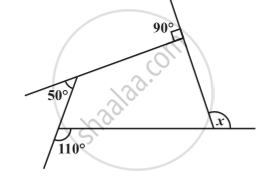Share

# Sum of the Measures of the Exterior Angles of a Polygon

#### notes

The sum of the measures of the external angles of any polygon is 360°.
For example :  Find measure x in following fig.Solution :
x + 90° + 50° + 110° = 360°
x + 250° = 360°
x = 110°

### Shaalaa.com

Sum of the Measures of the Exterior Angles of a Polygon [00:05:00]
S
0%

S